One property of the integral of the hyperbola

Let us consider the function defined by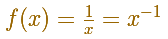Its graph is called a rectangular hyperbola.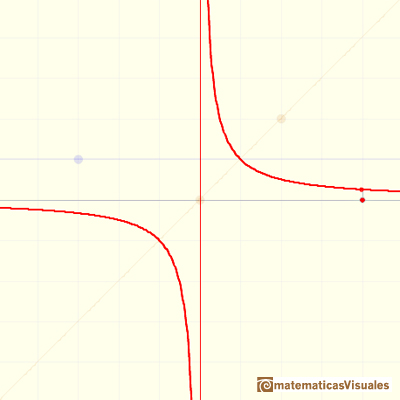Remember that of all the integrals of power functions the integral with exponent -1 was the only one which we cannot evaluate.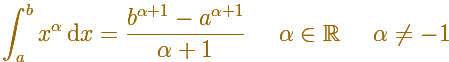The integral of power functions was know by Cavalieri from n=1 to n=9. Fermat was able to solve this problem using geometric progressions.

In what follows, a and b are two positive real numbers. These two numbers determine a curvilinear trapezoid, an area.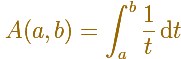If a and b are multiplied by the same positive number, then the new curvilinear trapezoid obtained .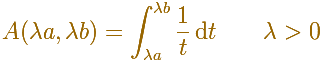has the same area as the originalUsing the applet, you can see this property. You can modify the limits of integration and clicking the play button you can see how the area is transforming continuously.

Some rectangles represent the areas.As the natural logaritm can be defined using the integral of the equilateral hiperbola, using this property you can justify that the logarithm of a product is the sum of the logarithms.

C.H.Edwards Jr. wrote: "The tables of Napier and Briggs and their followers revolutionized the art of numerical computation. However, the importance of logarithms in the historical development of the calculus stems from a discovery published in 1647 by the Belgian Jesuit Gregory St. Vincent, that implies a surprising connection between the natural logarithm function and the rectangular hyperbola xy = 1." (pag. 154)

In the next applet you can see a visual "demostration" of this property.

If you consider only one rectangle:Using more and more rectangles you can say that the two areas are the same.Now you can use this property to see that the logarithm of a product is the sum of the logarithms of the factors.

[Serge Lang, p.181]Let h be a positive number. Compare the area under the curve 1/x between 1 and 1+h and show that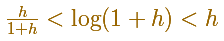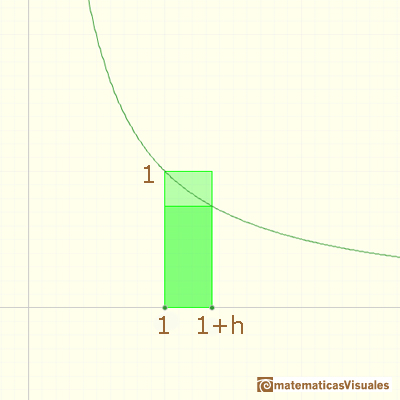Now, solve

Using a very similar argument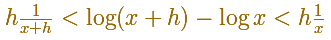Dividing both sides of our inequalities by the positive number h the inequalities are preserved, and we get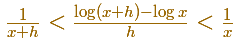As h approaches 0, our Newton quotient is squeezed and approaches 1/x.

This is the base of an argument to proveAlthough we are going to see more visual and intuitive approaches to the properties of the logarithm function I think that Serge Lang's comment at this point is interesting to consider: "From now on, we do not need our geometric intuition any more. The notion of area was used only to give us the existence of a function whose derivative is 1/x, and whose value at 1 is 0. All the arguments which follow depend on the fact that we have such a function." (Serge Lang, p. 177)

REFERENCES

A. I. Markushevich, Areas and Logarithms, D.C. Heath and Company, 1963.
Serge Lang, A First Course in Calculus, Third Edition, Addison-Wesley Publishing Company.
Tom M. Apostol, Calculus, Second Edition, John Willey and Sons, Inc.
Michael Spivak, Calculus, Third Edition, Publish-or-Perish, Inc.
Otto Toeplitz, The Calculus, a genetic approach, The University of Chicago Press, 1963.
Kenneth A. Ross, Elementary Analysis: The Theory of Calculus, Springer-Verlag New York Inc., 1980.
C.H. Edwards, Jr., The Historical Development of the Calculus, Springer-Verlag New York Inc., 1979.We can study several properties of exponential functions, their derivatives and an introduction to the number e.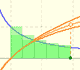The logarithm of the number e is equal to 1. Using this definition of the number e we can approximate its value.Constant e is the number whose natural logarithm is 1. It can be defined as a limit of a sequence related with the compound interest. Both definitions for e are equivalent.After the definition of the natural logarithm function as an integral you can define the exponential function as the inverse function of the logarithm.Different hyperbolas allow us to define different logarithms functions and their inversas, exponentials functions.Mercator published his famous series for the Logarithm Function in 1668. Euler discovered a practical series to calculate.By increasing the degree, Taylor polynomial approximates the exponential function more and more.The Complex Exponential Function extends the Real Exponential Function to the complex plane.The complex exponential function is periodic. His power series converges everywhere in the complex plane.The Fundamental Theorem of Calculus tell us that every continuous function has an antiderivative and shows how to construct one using the integral.The Second Fundamental Theorem of Calculus is a powerful tool for evaluating definite integral (if we know an antiderivative of the function).As an introduction to Piecewise Linear Functions we study linear functions restricted to an open interval: their graphs are like segments.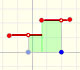A piecewise function is a function that is defined by several subfunctions. If each piece is a constant function then the piecewise function is called Piecewise constant function or Step function.A continuous piecewise linear function is defined by several segments or rays connected, without jumps between them.The integral of power functions was know by Cavalieri from n=1 to n=9. Fermat was able to solve this problem using geometric progressions.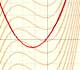If the derivative of F(x) is f(x), then we say that an indefinite integral of f(x) with respect to x is F(x). We also say that F is an antiderivative or a primitive function of f.The integral concept is associate to the concept of area. We began considering the area limited by the graph of a function and the x-axis between two vertical lines.Monotonic functions in a closed interval are integrable. In these cases we can bound the error we make when approximating the integral using rectangles.If we consider the lower limit of integration a as fixed and if we can calculate the integral for different values of the upper limit of integration b then we can define a new function: an indefinite integral of f.We can consider the polynomial function that passes through a series of points of the plane. This is an interpolation problem that is solved here using the Lagrange interpolating polynomial.